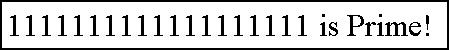The Repunit Primes Project

Curiosity

 11111 Repunit (1995 WL) is a main-belt asteroid discovered on November 16, 1995 by T. Kobayashi at Oizumi. In this video, you can see the Bob Speca's Dominos challenge. In the challenge Bob uses 111,111 dominoes.You can listen this repunit number from 0:34 to 0:43, in the timeline of the video. URL: http://www.youtube.com/watch?v=jSaO_mWbvGw&t=0m57s The ElektroSoft (http://www.elektrosoft.it), the Giovanni Di Maria's firm, was born on 11/11/11 at 11:11 o'clock. Nov 18, 2015 - 17:30 PM ET, ("Lucara" or the "Company"), the high quality diamond producer with assets in Botswana, is pleased to announce the recovery of a 1,111 carat gem quality. See the link. Jun 2020: Nice PRP number, made up of 50,473 digits, discovered by by Matt Stath: 66879*2*((10^2-1)/9)*((10^19-1)/9)*((10^23-1)/9)*((10^317-1)/9)*((10^1031-1)/9)*((10^49081-1)/9)+1 is PRP! 66879*R(2)*R(19)*R(23)*R(317)*R(1031)*R(49081)+1 is PRP! Jun 2020: Nice PRP number, made up of 13,564 digits, discovered by by Matt Stath: (10^13564-1)/9+(10^1031-1)/9+(10^317-1)/9+(10^23-1)/9+(10^19-1)/9+(10^2-1)/9+(10^1-1)/9 is PRP! R(13564)+R(1031)+R(317)+R(23)+R(19)+R(2)+R(1) is PRP! Some of The Repunits and Repunit factors with 10,000 or more digits are at http://www.primenumbers.net/prptop/searchform.php?form=%2810%5En-1%29%2F%3F&action=Search